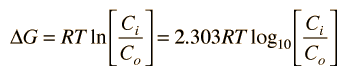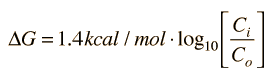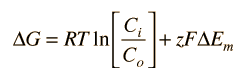# Energetics of Solute Diffusion

Diffusion is the process of transferring matter from an area of high concentration to an area of low concentration under the influence of thermal energy. For biological applications, it is important to evaluate diffusion of solute molecules, particularly across cell membranes. Diffusion always proceeds toward an equilibrium where the concentrations are equal, but life is always a non-equilibrium process and there are many situations where non-equilibrium concentrations of solute are maintained.

It is useful to know the energy required to maintain non-equilibrium concentrations, and in biological contexts this energy is usually expressed in terms of the Gibbs free energy. The change in free energy associated with the movement of an uncharged solute (a non-electrolyte) across a cell membrane can be expressed aswhere Ci and Co are the concentrations inside and outside the cell and R = 8.3145 J/mol = 1.987 cal/mole is the gas constant. It is common to use 25°C = 298.15K for biological reference calculations, and at that temperature this relationship becomesIf the solute is charged (an electrolyte), then the voltage difference between the inside and outside must be taken into account. The expression for free energy difference then becomeswhere z is the charge of the solute and F is the Faraday constant 23.06 kcal/V equivalent where an equivalent is the amount of the electrolyte having one mole of charge.

Index

Reference
Karp
Sec 4.7

 HyperPhysics***** Biology R Nave
Go Back